We all know about inverter - it is a device which converts DC into AC. And we previously learned about Different types of inverters and built a single phase 12v to 220v inverter.  A 3 Phase Inverter converts the DC voltage into 3 Phase AC supply. Here in this tutorial, we will learn about Three Phase Inverter and its working, but before going any further let us have a look at the voltage waveforms of the three-phase line. In the above circuit, a three-phase line is connected to a resistive load and the load draws power from the line. If we draw the voltage waveforms for each phase then we will have a graph as shown in the figure. In the graph, we can see three voltage waveforms are out of phase with each other by 120º.

In this article, we will discuss 3 Phase Inverter Circuit which is used as DC to 3 phase AC converter. Do remember that, even in the modern days achieving a completely sinusoidal waveform for varying loads is extremely difficult and is not practical. So here we will discuss the working of an ideal three-phase converter circuit neglecting all the issues related to practical 3 phase inverter.

### 3 Phase Inverter Working

Now let us look into the 3 Phase Inverter Circuit and its ideal simplified form.

Below is a three-phase inverter circuit diagram designed using thyristors & diode (for voltage spike protection)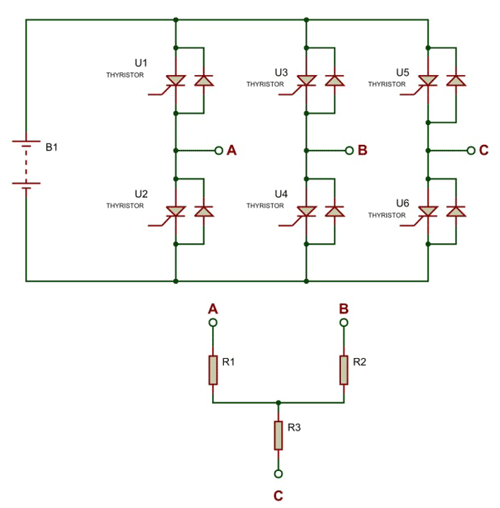And below is a three-phase inverter circuit diagram designed using only switches. As you can see this six mechanical switch setup is more useful in understanding the 3 phase inverter working than the cumbersome thyristor circuit.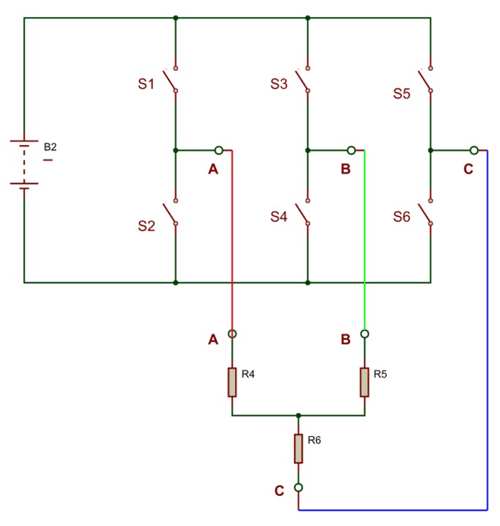What we will do here is open & symmetrically closes these six switches to get the three-phase voltage output for the resistive load. There are two possible ways for triggering the switches to achieve the desired result, one in which switches conduct for 180º and another in which switches only conduct for 120º. Let us discuss each pattern below:

### A) Three Phase Inverter- 180 Degree Conduction Mode

The ideal circuit is drawn before it can be divided into three segments namely segment one, segment two & segment three and we will use these notational in the later section of the article. Segment one consists of a pair of switches S1&S2, segment two consists of switching pair S3 &S4 and segment three consists of switching pair S5&S6. At any given time both the switches in the same segment should never be closed as it leads to battery short circuits failing the entire setup, so this scenario should be avoided at all times.

Now let’s start switching sequence by closing the switch S1 in the first segment of the ideal circuit and let’s name the start as 0º. Since the selected time of conduction is 180º the switch S1 will be closed from 0º to 180º.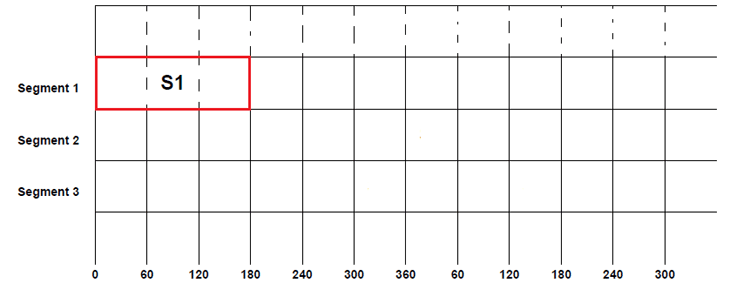But after 120º of the first phase, the second phase will also have a positive cycle as seen in the three-phase voltage graph, so switch S3 will be closed after S1. This S3 will also be kept closed for another 180º. So S3 will be closed from 120º to 300º and it will be open only after 300º.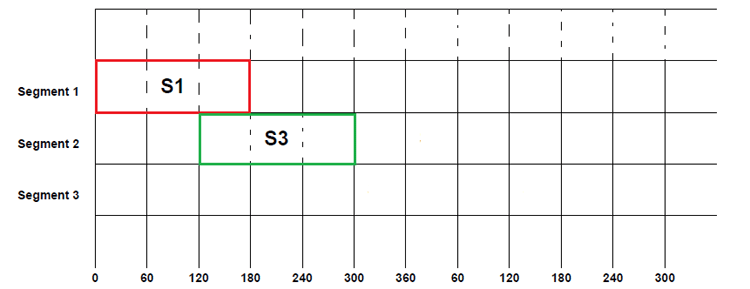Similarly, the third phase also has a positive cycle after 120º of second phase positive cycle, as shown in the graph at the beginning of the article. So the switch S5 will be closed after 120º S3 closing i.e. 240º. Once the switch is closed it will be kept closed for coming 180º before being opened, with that the S5 will be closed from 240º to 60º (second cycle).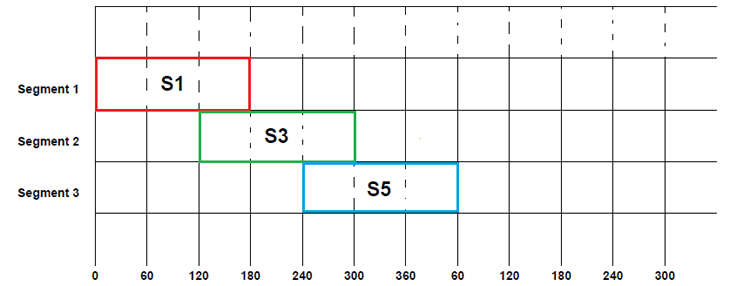Up until now, all we did was assume that’s the conduction is done once the top layer switches are closed but for current flow from the circuit must be completed. Also, do remember that both switches in the same segment should never be in the closed at the same time, so if one switch is closed then another must be open.

For satisfying the above both conditions, we will close S2, S4& S6 in a predetermined order. So only after S1 gets opened we will have to close S2. Similarly, S4 will be closed after S3 gets opened at 300º and in the same way S6 will be closed after S5 completes the conduction cycle. This cycle of switching between switches of the same segment can be seen below figure. Here S2 followsS1, S4 follows S3 and S6 follows S5.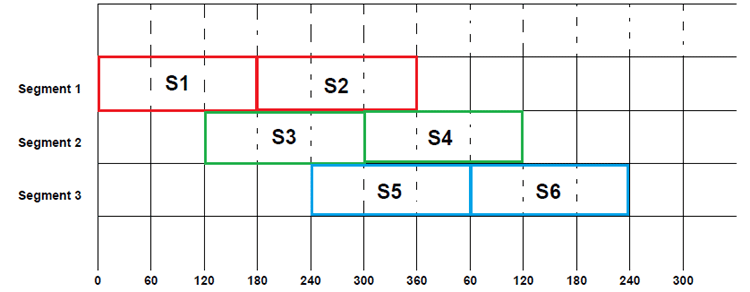By following this symmetrical switching we can achieve the desired three-phase voltage represented in the graph. If we fill in the beginning switching sequence in the above table we will have a complete switching pattern for 180º conduction mode as below.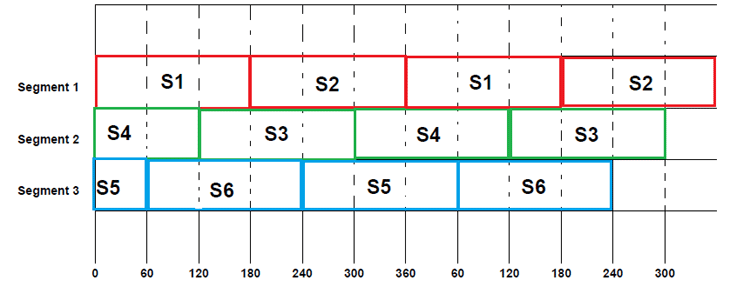From the above table we can understand that:

From 0-60: S1, S4 & S5 are closed and the remaining three switches are opened.

From 60-120: S1, S4 & S6 are closed and the remaining three switches are opened.

From 120-180: S1, S3&S6 are closed and the remaining three switches are opened.

And the sequence of switching goes on like that. Now let us draw the simplified circuit for each step to better understand the current flow and voltage parameters.

Step1: (for 0-60) S1, S4&S5 are closed while the remaining three switches are open. In such a case, the simplified circuit can be as shown below.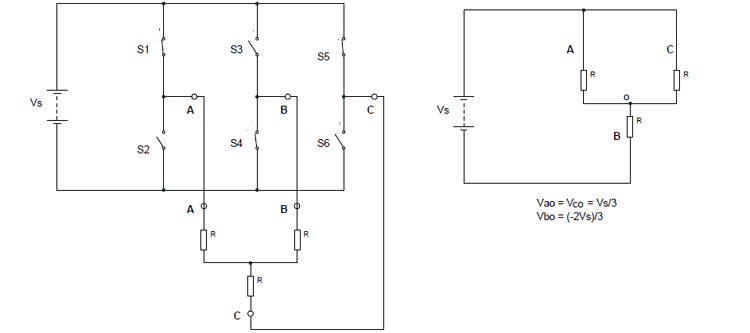So for 0 to 60: Vao = Vco= Vs/3 ; Vbo = -2Vs/3

By using these we can derive the line voltages as:

```Vab = Vao – V bo = Vs
Vbc = Vbo – Vco = -Vs
Vca = Vco – Vao = 0```

Step2: (for 60 to 120) S1, S4&S6 are closed while the remaining three switches are open. In such a case, the simplified circuit can be as shown below.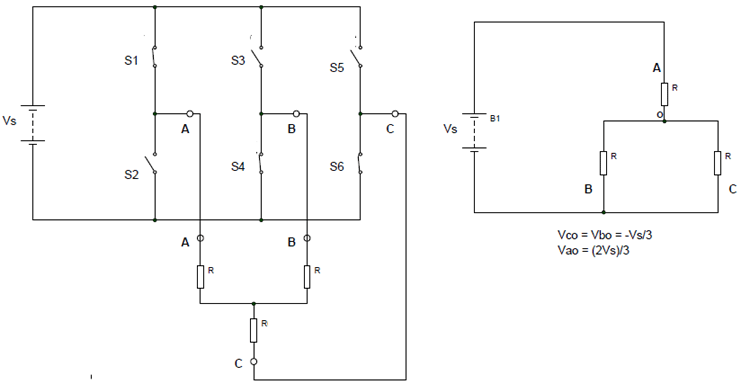So for 60 to 120: Vbo = Vco= -Vs/3 ; Vao = 2Vs/3

By using these we can derive the line voltages as:

```Vab = Vao – Vbo = Vs
Vbc = Vbo – Vco = 0
Vca = Vco – Vao = -Vs```

Step3: (for 120 to 180) S1, S3&S6 are closed while the remaining three switches are open. In such a case, the simplified circuit can be drawn as below.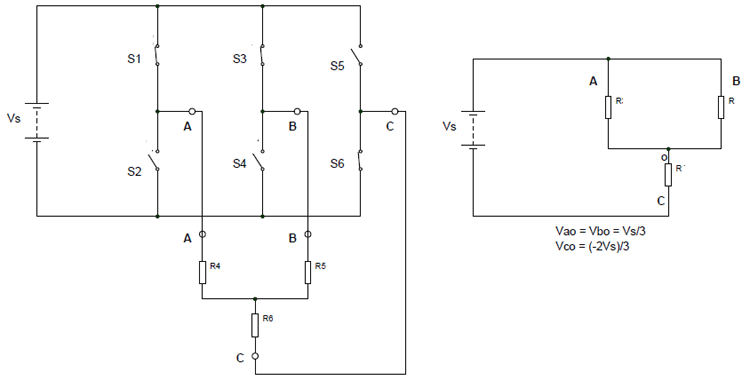So for 120 to 180: Vao = Vbo= Vs/3 ; Vco = -2Vs/3

By using these we can derive the line voltages as:

```Vab = Vao – V bo = 0
Vbc = Vbo – Vco = Vs
Vca = Vco – Vao = -Vs```

Similarly, we can derive the phase voltages and line voltages for the next steps in the sequence. And it can be shown as the figure given below: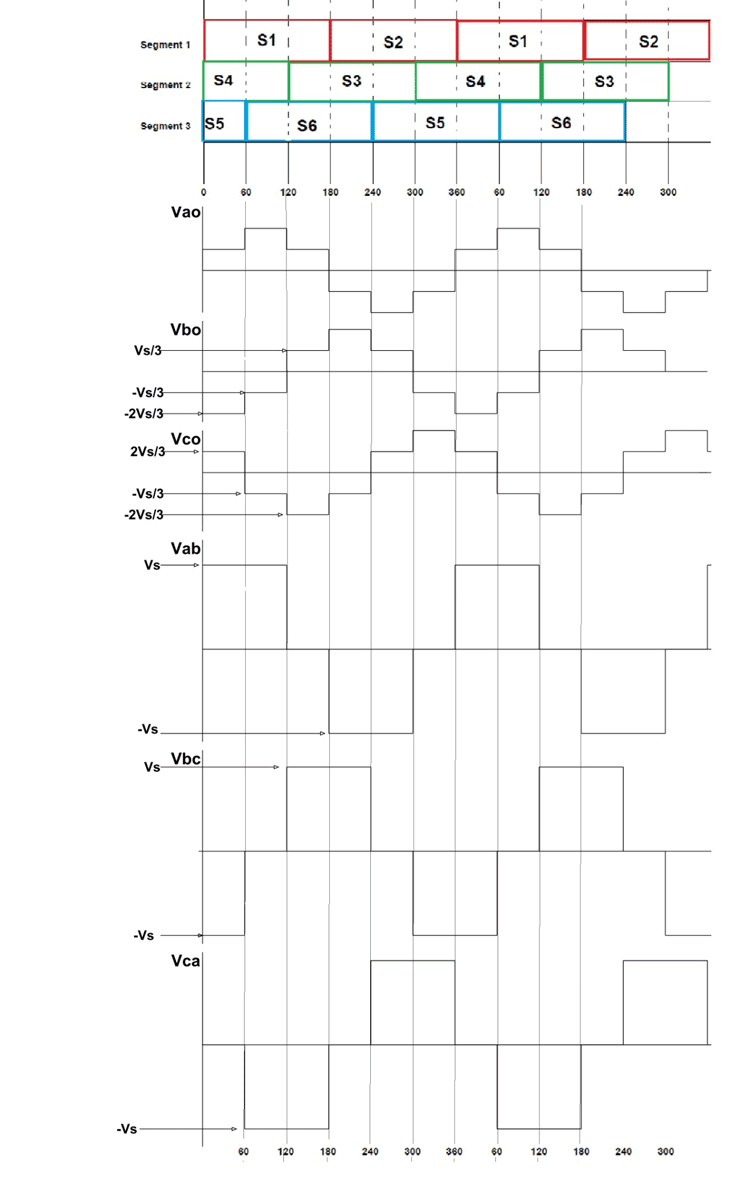### A) Three Phase Inverter- 120 Degree Conduction Mode

The 120º mode is similar to 180º at all aspects except the closing time of each switch is reduced to 120, which were 180 before.

As usual, let’s start switching sequence by closing the switch S1 in the first segment and be the start number to 0º. Since the selected time of conduction is 120º the switch S1 will be opened after 120º, so the S1 was closed from 0º to 120º.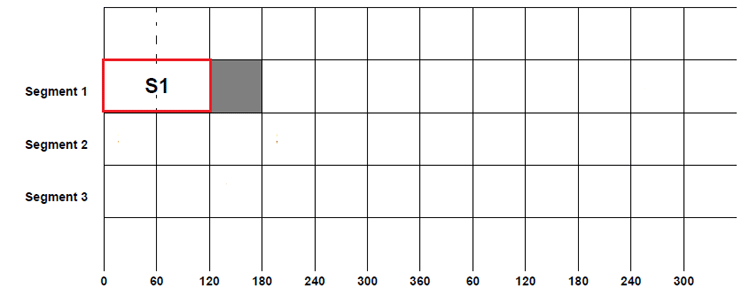Since half cycle of the sinusoidal signal goes from 0 to 180º, for the remaining time S1 will be open and is represented by the gray area above.

Now after 120º of the first phase, the second phase will also have a positive cycle as mentioned before, so switch S3 will be closed after S1. This S3 will also be kept closed for another 120º. So S3 will be closed from 120º to 240º.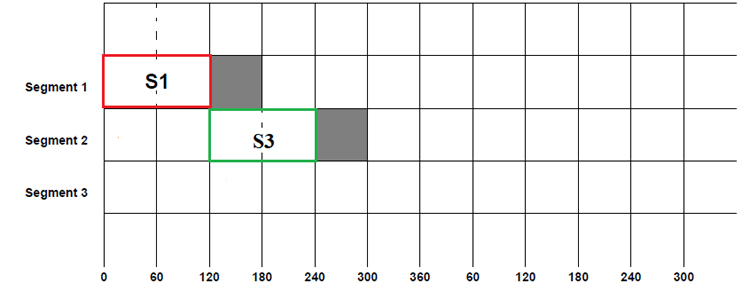Similarly, the third phase also has a positive cycle after 120º of the second phase positive cycle so the switch S5 will be closed after 120º of S3 closing. Once the switch is closed, it will be kept closed for coming 120º before being opened and with that, the switch S5 will be closed from 240º to 360º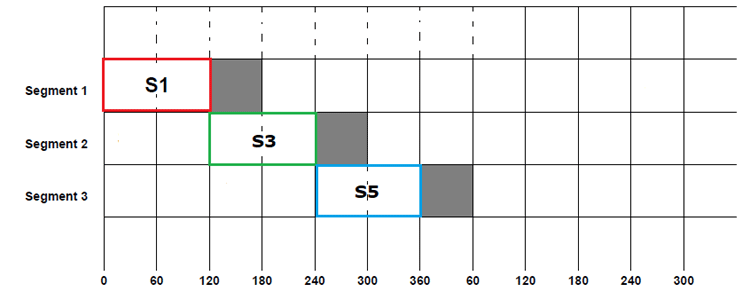This cycle of symmetrical switching will be continued for achieving the desired three-phase voltage. If we fill in the beginning and ending switching sequence in the above table we will have a complete switching pattern for 120º conduction mode as below.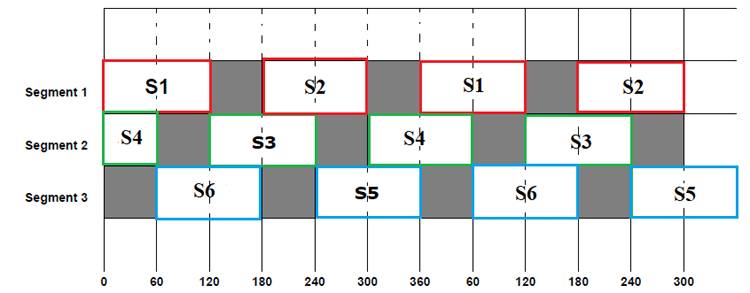From the above table we can understand that:

From 0-60: S1&S4 are closed while remaining switches are opened.

From 60-120: S1 &S6 are closed while remaining switches are opened.

From 120-180: S3&S6 is closed while remaining switches are opened.

From 180-240: S2&S3 are closed while remaining switches are opened

From 240-300: S2&S5 are closed while remaining switches are opened

From 300-360: S4&S5 are closed while remaining switches are opened

And this sequence of steps goes on like that. Now let us draw the simplified circuit for each step to better understand the current flow and voltage parameters of the 3 Phase Inverter circuit.

Step1: (for 0-60) S1, S4 are closed while the remaining four switches are open. In such a case, the simplified circuit can be shown as below.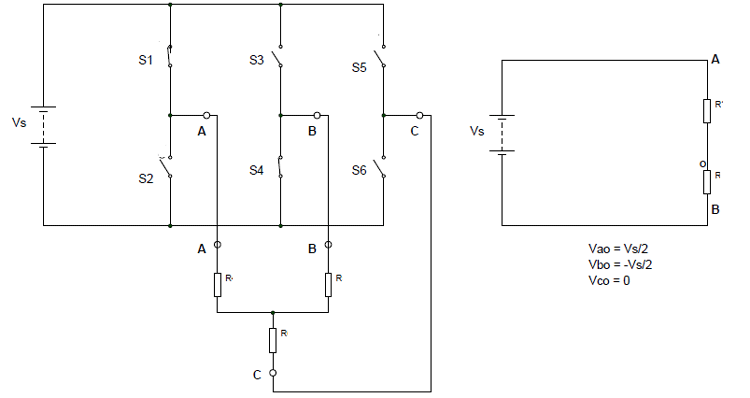So for 0 to 60: Vao = Vs/2, Vco= 0 ; Vbo = -Vs/2

By using these we can derive the line voltages as:

```Vab = Vao – V bo = Vs
Vbc = Vbo – Vco = -Vs/2
Vca = Vco – Vao = -Vs/2```

Step2: (for 60 to 120) S1 &S6 are closed while the remaining switches are open. In such a case, the simplified circuit can be shown as below.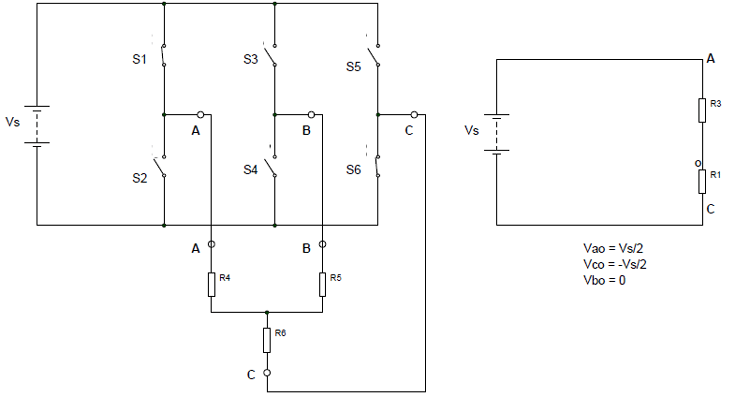So for 60 to 120: Vbo =0, Vco= -Vs/2 & Vao = Vs/2

By using these we can derive the line voltages as:

```Vab = Vao – Vbo = Vs/2
Vbc = Vbo – Vco = Vs/2
Vca = Vco – Vao = -Vs```

Step3: (for 120 to 180) S3&S6 are closed while the remaining switches are open. In such a case, the simplified circuit can be shown as below.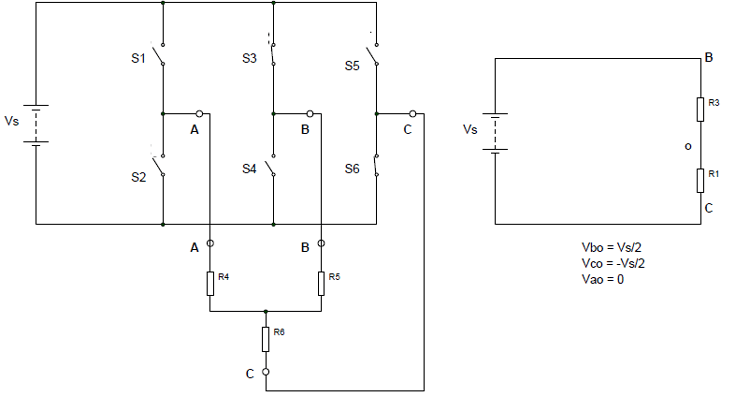So for 120 to 180: Vao =0, Vbo= Vs/2 & Vco = -Vs/2

By using these we can derive the line voltages as:

```Vab = Vao – V bo = -Vs/2
Vbc = Vbo – Vco = Vs
Vca = Vco – Vao = -Vs/2```

Similarly, we can derive the phase voltages and line voltages for the next upcoming steps. And if we draw a graph for all the steps then we will get something like below.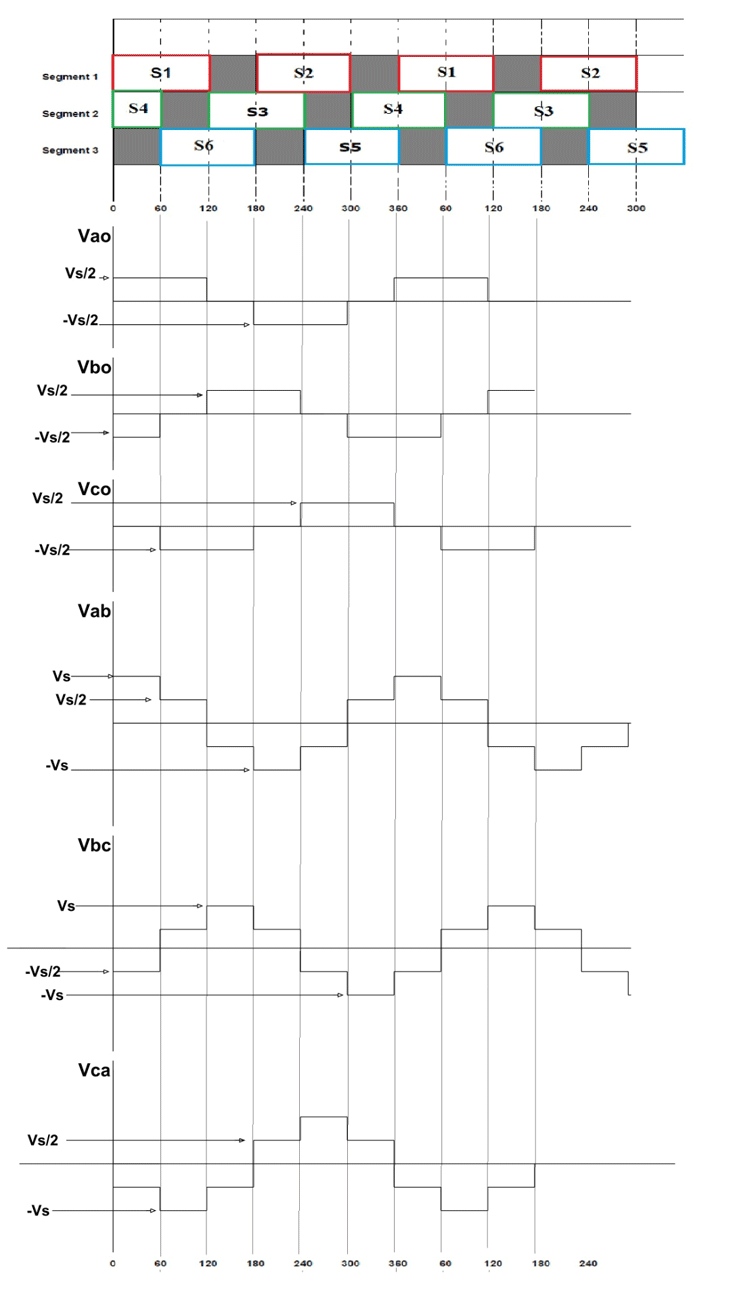It can be seen in the output graphs of both 180º and 120º switching cases that we have achieved an alternating three-phase voltage at the three output terminals. Although the output waveform is not a pure sine wave, it did resemble the three-phase voltage waveform. This is a simple ideal circuit and approximated waveform for understanding 3 phase inverter working. You can design a working model based on this theory using thyristors, switching, control, and protection circuitry.